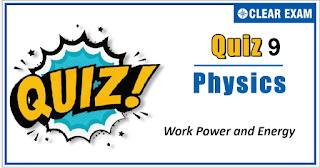## [LATEST]\$type=sticky\$show=home\$rm=0\$va=0\$count=4\$va=0

As per analysis for previous years, it has been observed that students preparing for NEET find Physics out of all the sections to be complex to handle and the majority of them are not able to comprehend the reason behind it. This problem arises especially because these aspirants appearing for the examination are more inclined to have a keen interest in Biology due to their medical background.

Furthermore, sections such as Physics are dominantly based on theories, laws, numerical in comparison to a section of Biology which is more of fact-based, life sciences, and includes substantial explanations. By using the table given below, you easily and directly access to the topics and respective links of MCQs. Moreover, to make learning smooth and efficient, all the questions come with their supportive solutions to make utilization of time even more productive. Students will be covered for all their studies as the topics are available from basics to even the most advanced.

Q1. If the unit of force and length each be increased by four times, then the unit of energy is increased by
•  16 times
•  8 times
•  2 times
•  4 times
Solution
(a) Work = Force × Displacement (length) If unit of force and length be increased by four times then the unit of energy will increase by 16 times

Q2.A billiard ball moving with a speed of 5 m/s collides with an identical ball originally at rest. If the first ball stops after collision, then the second ball will move forward with a speed of
•  10 ms-1
•  5 ms-1
•  2.5 ms-1
•  Ka1.0 ms-1
Solution
(b) In elastic head on collision velocities gets interchanged

Q3.  A body of mass 5 kg is thrown vertically up with a kinetic energy of 490 J. The height at which the kinetic energy of the body becomes half of the original value is
•  12.5 m
•  10 m
•  2.5 m
•  5 m
Solution
(d) According to law of conservation of energy 1/2 mu2=1/2 mv2+mgh 490=245+5×9.8×h h=245/49=5m

Q4. A 0.5 kg ball is thrown up with an initial speed 14 m/s and reaches a maximum height of 8.0m. How much energy is dissipated by air drag acting on the ball during the ascent
•  19.6 Joules
•  4.9 Joules
•  10 Joules
•  9.8 Joules
Solution
(d) If there is no air drag then maximum height H=u2/2g=(14×14)/(2×9.8)=10 m But due to air drag ball reaches up to height 8m only. So loss in energy =mg(10-8)=0.5×9.8×2=9.8 J

Q5.A particle is released from a height s. At certain height its kinetic energy is three times its potential energy. The height and speed of the particle at that instant are respectively
•  s/4,3gs/2
•  s/4,√3gs/2
•  s/2,√3gs/2
•  s/4,√3gs/4
Solution
(d) Velocity at B when dropped from A where AC=s v2=u2+2g(s-x) ….(i)
v2=2g(s-x) ….(ii)
Potential energy at B=mgx ∴ Kinetic energy = 3× potential energy 1/2 m×2g(s-x)=3×mgx or (s-x)=3x or s=4x or x=s/4 From Eq. (i) v2=2g(s-x) =2g(s-s/4) =(2g×3s)/4=3gs/2 ∴ x=s/4 and v=√(3gs/2)

Q6. A ball of mass 2 kg and another of mass 4 kg are dropped together from a 60 ft tall building .After a fall of 30 ft each towards earth ,their respective kinetic energies will be in the ratio of
•  √2:1
•  1.4
• 1.2
•  1:√2
Solution
(c) Kinetic energy = 1/2 mv^2 As both balls are falling through same height, therefore they possess same velocity. ∴ (KE)11/(KE)2=m1/m2=2/4=1/21

Q7.When a spring is stretched by 2 cm, it stores 100 J of energy. If it is stretched further by 2 cm, the stored energy will be increased by
•  100 J
•  20 J
•  300 J
•  400 J
Solution
(c) 100= 1/2 kx2 [Given] W=1/2 k(x22-x_12 )=1/2 k[(2x)2-x2 ] =3×(1/2 kx2 )=3×100=300 J

Q8.A body of mass 2 kg is projected at 20ms-1 at an angle 60° above the horizontal. Power Due to the gravitational force at its heights point is
•  200 W
•  100√3 W
•  50 W
•  Zero W
Solution
(a) Given, m=2kg, v=20ms-1,θ=60° Power(P)is given as P=F∙v=Fv cos⁡θ P=mgv cos⁡θ ∴ P=2×20×10×cos⁡〖60°〗 P=2×20×10×1/2 ⇒ P=200 W

Q9.A 10kg mass moves along x-axis. Its acceleration as a function of its position is shown in the figure. What is the total work done on the mass by the force as the mass moves from x=0 to x=8 cm
•  8×10-2joules
•  16×10-2joules
•  4×10-4joules
•  1.6×10-3 joules
Solution
(a) Work done = Area covered in between force displacement curve and displacement axis = Mass ×Area covered in between acceleration-displacement curve and displacement axis =10×1/2 (8×10-2×20×10-2)=8×10-2 J

Q10. A shell of mass m moving with velocity v suddenly breaks into 2 pieces. The part having mass m/4 remains stationary. The velocity of the other shell will be
•  v
•  2v
•  3/4v
• 4/3v
Solution
(d) According to conservation of momentum
mv=(m/4) v1+(3m/4) v22⇒4/3 v#### Written by: AUTHORNAME

AUTHORDESCRIPTION## Want to know more

Please fill in the details below:

## Latest NEET Articles\$type=three\$c=3\$author=hide\$comment=hide\$rm=hide\$date=hide\$snippet=hide

Name

ltr
item
BEST NEET COACHING CENTER | BEST IIT JEE COACHING INSTITUTE | BEST NEET, IIT JEE COACHING INSTITUTE: Work-Power and Energy-Quiz-9
Work-Power and Energy-Quiz-9
https://1.bp.blogspot.com/-HcjReO0wIL4/X5O4MCa467I/AAAAAAAAFNE/nsI1SAbTwJIXBxDa5d4ai_vCdVsKWjisQCLcBGAsYHQ/s320/Screenshot%2B2020-10-24%2B104258.png
https://1.bp.blogspot.com/-HcjReO0wIL4/X5O4MCa467I/AAAAAAAAFNE/nsI1SAbTwJIXBxDa5d4ai_vCdVsKWjisQCLcBGAsYHQ/s72-c/Screenshot%2B2020-10-24%2B104258.png
BEST NEET COACHING CENTER | BEST IIT JEE COACHING INSTITUTE | BEST NEET, IIT JEE COACHING INSTITUTE
https://www.cleariitmedical.com/2020/10/work-power-and-energy-quiz-9-dear.html
https://www.cleariitmedical.com/
https://www.cleariitmedical.com/
https://www.cleariitmedical.com/2020/10/work-power-and-energy-quiz-9-dear.html
true
7783647550433378923
UTF-8